# 802.11az Indoor Positioning Using Time of Arrival

This example shows how to calculate the position of a station (STA) within an IEEE® 802.11az™ Wi-Fi™ network using the time of arrival (TOA) positioning approach in conjunction with the trilateration technique. The example measures the positioning accuracy with respect to the signal-to-noise ratio (SNR) by computing the distance between the estimated and the actual STA positions in an additive white Gaussian noise (AWGN) channel.

### Introduction

The emerging 802.11az standard , commonly referred to as next generation positioning (NGP), enables a STA to identify its position relative to other STAs. This standard supports two high-efficiency (HE) ranging physical layer (PHY) protocol data unit (PPDU) formats:

• HE ranging null data packet (NDP)

• HE trigger-based (TB) ranging NDP

The HE ranging NDP and HE TB ranging NDP are the respective analogues of the HE sounding NDP and HE TB NDP feedback PPDU formats, as defined in the 802.11ax™ standard. For more information on these HE PPDU formats, see .

The HE ranging NDP supports the positioning of one or more users with an optional secure HE long training field (HE-LTF) sequence. The single-user HE ranging waveform contains HE-LTF symbols for a single user, which also support an optional secure HE-LTF sequence. The multi-user HE ranging waveform permits only secure HE-LTF symbols for multiple users. To improve position estimation accuracy, single-user and multi-user waveforms can contain multiple repetitions of the HE-LTF symbols. To parameterize and generate 802.11az HE ranging NDPs, see 802.11az Waveform Generation.

This example simulates an 802.11az network consisting of a STA and multiple access points (APs). To estimate the position of a STA, the network requires a minimum of three APs. The example models the ranging measurement exchange between the STA and each AP by:

• Generating an HE ranging NDP

• Adding a delay to account for the propagation over distance

• Adding AWGN to the transmitted NDP to create a desired SNR

• Performing synchronization and channel estimation at the receiver and,

• Computing the round trip time (RTT) from the phase shifts in the frequency-domain channel estimates

Estimate the position of the STA by performing trilateration technique using the distances derived from the RTT. At each SNR point, determine the average position accuracy by comparing the estimated position of STA to its actual position over multiple iterations. The SNR is the same for all the links between STA and APs.

This diagram illustrates a network with a STA and multiple APs.

802.11az Wi-Fi Network### Ranging Measurement Exchange

In this example, the STA acts as an initiating station (ISTA) and APs act as the receiving stations (RSTA). Between the APs (RSTAs) and STA (ISTA), the non-TB ranging measurement exchange sequence occurs in two phases:

1. Measurement sounding phase

2. Measurement reporting phase

The STA and AP exchange the ranging NDP announcement (NDPA) frame and HE ranging NDP frames (I2R/R2I NDP) during the measurement sounding phase and location measurement report (LMR) frames during the measurement reporting phase shown in this figure.

Non-TB ranging measurement exchange sequenceThis example simulates the measurement sounding exchange between the APs and STA to estimate the position of the STA.

#### Measurement Sounding Phase

The STA and APs perform the TOA measurements by capturing the timestamps of the NDP. The STA records the time (t1) at which the uplink NDP (UL NDP) is transmitted. The AP then captures the time (t2) at which the UL NDP arrives and records the time (t3) at which the downlink NDP (DL NDP) is transmitted. The STA finally captures the time (t4) at which the DL NDP arrives.

The RTT is defined as:

RTT = (t4 – t1) – (t3 – t2)

This diagram illustrates the measurement sounding phase between a STA and a single AP.

Timing diagram of a Measurement Sounding phase in non-TB RangingIn this example, to compute RTT, use a phase-shift-based timing estimates tp2 and tp4 instead of the time stamps based TOAs, t2 and t4. Determine tp2 and tp4 from the phase slope of the frequency domain channel estimates of the corresponding NDP.

The phase shifts based RTT is defined as:

RTT = (t4 – t1) – (t3 – t2’’), with t2’’ = tp2 – (tp4 – t4)

Compute the distance between the STA and each AP from the RTT as:

distance = RTT*c/2, where c is speed of light

Use the trilateration technique to estimate the position of STA from the estimated distances and the known position of APs.

### Simulation Parameters

Specify the number of APs in a network. To estimate the position of a STA, the network requires a minimum of three APs.

`numAPs =3; % Number of APs, must be >=3`

Specify the SNR range and the number of iterations to simulate each SNR point.

```snr =5:2:15; % SNR (dB) numIterations =100; % Number of iterations to average the position error```

### Waveform Configuration

To configure HE ranging NDP parameters corresponding to the APs and STA, use the `heRangingConfig` object.

```chanBW ='CBW20'; % Channel bandwidth numTx =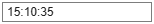1; % Number of transmit antennas numSTS =1; % Number of space-time streams numLTFRepetitions =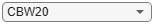2; % Number of LTF repetitions % Configure the HE ranging NDP parameters of the STA cfgSTA = heRangingConfig; cfgSTA.ChannelBandwidth = chanBW; cfgSTA.NumTransmitAntennas = numTx; cfgSTA.SecureHELTF = true; cfgSTA.User{1}.NumSpaceTimeStreams = numSTS; cfgSTA.User{1}.NumHELTFRepetition = numLTFRepetitions; cfgSTA.GuardInterval = 1.6; % Configure the HE ranging NDP parameters of the APs cfgAP = cell(1,numAPs); for iAP = 1:numAPs cfgAP{iAP} = heRangingConfig; cfgAP{iAP}.ChannelBandwidth = chanBW; cfgAP{iAP}.NumTransmitAntennas = numTx; cfgAP{iAP}.SecureHELTF = true; cfgAP{iAP}.User{1}.NumSpaceTimeStreams = numSTS; cfgAP{iAP}.User{1}.NumHELTFRepetition = numLTFRepetitions; cfgAP{iAP}.GuardInterval = 1.6; end```

### Ranging Measurement and Position Estimation

This example estimates the STA position from the ranging measurement exchange between the STA and the APs by following these steps:

1. Position the STA at an origin and `numAPs` APs randomly in the xy-plane.

2. Estimate the distance by modeling the measurement exchange for each STA-AP and AP-STA link: Generate a ranging NDP and delay the NDP according to the distance, and add AWGN. Perform synchronization on the received waveform and estimate the channel. Compute the transmission time from the channel estimates.

3. Compute the RTT from the transmission times, and estimate the distance between the STA and each AP.

4. Estimate the STA position by performing trilateration using the estimated distances.

Repeat the above process for `numIteration` times with random AP positions over a range of SNR points.

This figure illustrates the processing for each link between the STA and an AP.

Example workflow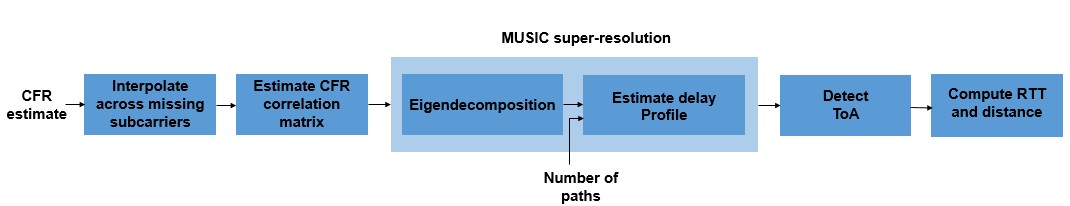```% Validate the simulation parameters if numAPs<3 error('Number of APs must be greater than or equals to 3'); end if numIterations<=0 error('Number of iterations must be a positive integer scalar'); end % Define constants speedOfLight = physconst('lightspeed'); % Speed of light (m/s) subcarrierSpacing = 78.125e3; % Subcarrier spacing (Hz) carrierFrequency = 5e9; % Carrier frequency (Hz) if carrierFrequency == 5e9 delayULDL = 16e-6; % Time gap (tDelay) between UL NDP TOA and DL NDP TOD (sec) elseif carrierFrequency == 2.4e9 delayULDL = 8e-6; end % Get sample rate sampleRate = heRangingSampleRate(cfgSTA); % Get occupied subcarrier indices and OFDM parameters ofdmInfo = wlanHEOFDMInfo('HE-LTF',chanBW,cfgSTA.GuardInterval); % Initialize average position error numSNR = numel(snr); % Number of SNR points avgPositionError = zeros(1,numSNR); for isnr = 1:numSNR % Loop over SNR range % Set random substream index to ensure that each iteration uses a % repeatable set of random numbers stream = RandStream('combRecursive','Seed',123456); stream.Substream = isnr; RandStream.setGlobalStream(stream); % Loop over number of iterations to average the positioning error. For % each iteration, generate random positions for the STA and APs and model % ranging measurement exchange between each AP and STA. positionError = 0; linkFailCount = 0; for iter = 1:numIterations % Randomly place the STA and APs in the xy-plane [positionSTA, positionAP, distance] = heGeneratePositions(numAPs); % Range-based delay delay = distance/speedOfLight; sampleDelay = delay*sampleRate; % Loop over the number of APs distEst = zeros(1,numAPs); % Estimate distance for ap = 1:numAPs linkType = ["Uplink", "Downlink"]; % Time of departure of UL NDP (t1) todUL = randsrc(1,1,0:1e-9:1e-6); % Loop for both UL and DL transmission numLinks = numel(linkType); txTime = zeros(1,numLinks); for l = 1:numLinks if linkType(l) == "Uplink" % STA to AP cfgSTA.UplinkIndication = 1; % For UL % Generate a random secure HE-LTF sequence for the exchange cfgSTA.User{1}.SecureHELTFSequence = dec2hex(randsrc(1,10,(0:15)))'; cfg = cfgSTA; else % AP to STA % Generate a random secure HE-LTF sequence for the exchange cfgAP{ap}.User{1}.SecureHELTFSequence = dec2hex(randsrc(1,10,(0:15)))'; cfg = cfgAP{ap}; % For DL end % HE Ranging NDP transmission tx = heRangingWaveformGenerator(cfg); % Introduce time delay in the transmit waveform txDelay = heDelaySignal(tx,sampleDelay(ap)); % Add both integer and fractional delay % Pass the waveform through AWGN channel % Account for noise energy in nulls so the SNR is defined % per active subcarrier and per transmit antenna rx = awgn(txDelay,snr(isnr)-10*log10(ofdmInfo.FFTLength/(numTx*ofdmInfo.NumTones))); % Perform synchronization by assuming that the transmit % side secure HE-LTF sequence is known at the receiver [chanEst, integerOffset] = heRangingSynchronize(rx,cfg); % Estimate the transmission time between UL and DL if ~isempty(chanEst) % If packet detection is successful diffAngleChan = diff(unwrap(angle(chanEst))) ; fracDelay = -mean(diffAngleChan(:))/(2*pi*subcarrierSpacing); % Estimate fractional time delay intDelay = integerOffset/sampleRate; % Estimate integer time delay txTime(l) = intDelay + fracDelay; % Transmission time rangeEstFlag = 1; % If packet detection is successful, enable the range estimation flag else rangeEstFlag = 0; % If packet detection fails, disable the range estimation flag break; end end if rangeEstFlag % If packet detection is successful % TOA of UL waveform (t2) toaUL = todUL + txTime(1); % Time of departure of DL waveform (t3) todDL = toaUL + delayULDL; % TOA DL waveform (t4) toaDL = todDL + txTime(2); % Compute the RTT rtt = (toaDL-todUL) - (todDL-toaUL); % Estimate the distance between the STA and AP distEst(ap) = (rtt/2)*speedOfLight; else break; % If packet detection fails, discard the link between STA and AP end end % If range estimation flag is enabled, then estimate the STA % position using trilateration and compute the position error. if rangeEstFlag positionSTAEst = hePositionEstimate(positionAP,distEst); positionError = positionError + sqrt(sum((positionSTAEst - positionSTA).^2)); else positionSTAEst = NaN; linkFailCount = linkFailCount + 1; % Count the failed links used to average the position error end end if linkFailCount == numIterations % If all the links fail at a given SNR value disp(['At SNR = ',num2str(snr(isnr)),' dB, ', 'all ranging NDP packet transmissions failed']) else avgPositionError(isnr) = positionError/(numIterations-linkFailCount); disp(['At SNR = ',num2str(snr(isnr)),' dB, ', 'Positioning error in meters = ', num2str(avgPositionError(isnr))]) end end```
```At SNR = 5 dB, Positioning error in meters = 1.4137 At SNR = 7 dB, Positioning error in meters = 0.77532 At SNR = 9 dB, Positioning error in meters = 0.63 At SNR = 11 dB, Positioning error in meters = 0.51577 At SNR = 13 dB, Positioning error in meters = 0.42353 At SNR = 15 dB, Positioning error in meters = 0.37412 ```

### Simulation Results

When ranging NDP packet transmissions are successful, this section generates these plots:

Plot #1: Plot the STA, estimated STA and APs positions in the xy-plane with trilateration circles for the last SNR value and for the last iteration.

Plot #2: Plot the average position error (meters) against SNR (dB).

```if ~isnan(positionSTAEst) % Plot the position of APs and STA heRangingPlotPositions(positionAP,positionSTAEst,distEst,snr(isnr),iter); end```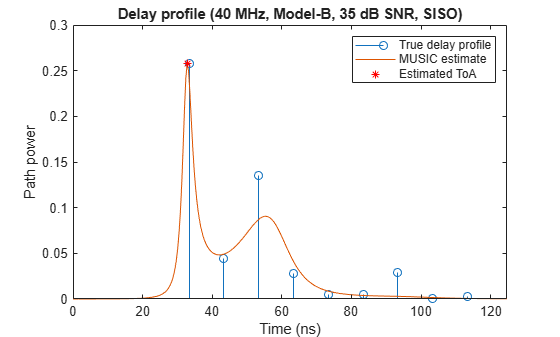```% Plot the position error (meters) against SNR (dB) figure semilogy(snr,avgPositionError,'-b*','LineWidth',2,... 'MarkerEdgeColor','b',... 'MarkerSize',10) hold on; grid on xlabel('SNR (dB)') ylabel('Estimated position error (meters)') title('Position accuracy in an 802.11az network')```### Conclusion

This example performs the HE ranging NDP measurement exchange between the APs and the STA to estimate the position of the STA using trilateration. The example also measures the positioning accuracy related to the SNR by computing the distance between the estimated and actual STA positions in an AWGN channel. To parameterize and generate 802.11az HE ranging NDPs, see 802.11az Waveform Generation.

### Further Exploration

To obtain a meaningful average position error, increase the number of iterations `numIterations` at each SNR point. To visualize the impact on position accuracy, you can also increase the number of LTF repetitions, `numLTFRepetitions` at both the STA and AP. This figure is generated by running a longer simulation with three APs, 1000 iterations for a channel bandwidth of 20 MHz, and a range of SNR values, 7:2:21. This plot shows that the estimated position error decreases with an increase in LTF repetitions.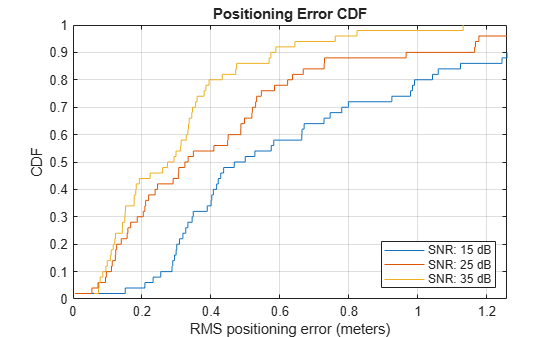### Selected Bibliography

1. IEEE P802.11az™/D2.0 Draft Standard for Information technology— Telecommunications and information exchange between systems Local and metropolitan area networks— Specific requirements - Amendment 3: Enhancements for positioning.

2. IEEE P802.11ax™/D4.1 Draft Standard for Information technology - Telecommunications and information exchange between systems - Local and metropolitan area networks - Specific requirements - Part 11: Wireless LAN Medium Access Control (MAC) and Physical Layer (PHY) Specifications - Amendment 6: Enhancements for High Efficiency WLAN.

## SupportGet trial now